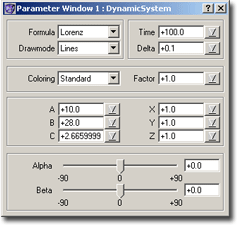### Dynamic Systems – ParametersFig.: Parameter Window

• Formula
At the moment ChaosPro offers you the following types:
• Lorenz
This is the basic Lorenz attractor. It is defined by the following 3 differential equations:
dx/dt=-ax+ay
dy/dt=cx-y-xz
dz/dt=-bz+xy
• Lorenz 1
That’s another attractor from Lorenz, similar to the previous one:
len=sqrt(x^2+y^2)
dx/dt=(-a-1) x + (a-b) y + (1-a) len + y z
dy/dt=(b-a) x – (a+1) y + (b+a) len – x z – len z
dz/dt=(0.5 y) – c z
• Lorenz 3
This Lorenz attractor has three loops:
len=sqrt(x^2+y^2)
dx=(-(a+1) x + (a-b+z) y)/3 + ((1-a) (x^2-y^2) + 2 (b+a-z) x y)/(3 len)
dy=((b-a-z) x – (a+1) y)/3 + (2 (a-1) x y + (b+a-z) (x^2-y^2))/(3 len)
dz=(3 x^2 y-y^3)/2 – cz
• Lorenz 4
And this attractor from Mr. Lorenz has four loops:
dx=(-a x^3 + (2 a+b-z) x^2 y + (a-2) x y^2 + (z-b) y^3) / (2 (x^2+y^2))
dy=((b-z) x^3 + (a-2) x^2 y + (-2 a-b+z) x y^2 – a y^3) / (2 (x^2+y^2))
dz=(2 x^3 y – 2 x y^3 – c z)
• Roessler:
That’s the attractor from Mr. Roessler, defined as follows:
dx/dt=-y-z
dy/dt=x+ay
dz/dt=b+xz-cz
• Drawmode
There are 2 possibilities: Points or Lines. These two modes draw the attractor starting at time 0 upto the defined time. The first mode Points draws only single points, which makes it look more clearly, the second mode Lines draws a line between the last point and the new one.
• Time / Delta
These two parameters define how many iterations are executed. The time starts at 0 and in each iteration cycle is increased by ‘Delta’ until ‘Time’ is reached. Because ChaosPro internally uses step size optimization, the parameter Delta only defines the starting value and an average step size (and, no, you don’t need to understand this…)
• Coloring
Standard: Uses a grey color to draw the attractor
Fixed: Uses the color at index 0 to draw the attractor
Time: Colors pixels according to the time
Position: Colors pixels according to their position in the space.
• A, B and C
Here you can set 3 parameter used in conjunction with the dynamic systems. I recommend to change the values only slightly, because the system could react to little changes.
• X, Y and Z
In the previous chapter (where I tried to explain some theoretical background) I mentioned, that independently from the starting point this point is always attracted by an object, which is called strange attractor due to its complicated structure. Everybody, who doesn’t believe this, has now the possibility to check this out and to change the starting point.
• Alpha and Beta
Because the Lorenz / Roessler-attractors are three dimensional objects, there is a little problem: How should one define the display area? In this program this is solved as follows: You define a two dimensional area just as if you were looking at the attractor from the front side. In order to not only view the attractor from the front, but from any point in the room, you then can change the view angles. There are two angles, which define the exact position of the observer: Alpha lets you define the degree of longitude, whereas Beta represents the degree of latitude.